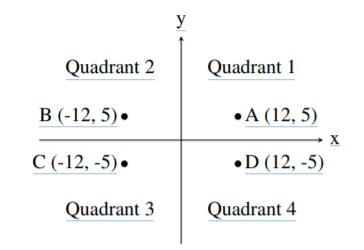TORONTO KIDS COMPUTER CLUB | Tuesday Python Homework 18:30 – 21.11.16
20088

# Tuesday Python Homework 18:30 – 21.11.16

## 21 Nov Tuesday Python Homework 18:30 – 21.11.16

Question:
A common problem in mathematics is to determine which quadrant a given point lies in. There are four quadrants, numbered from 1 to 4, as shown in the diagram below:For example, the point A, which is at coordinates (12, 5) lies in quadrant 1 since both its x and y values are positive, and point B lies in quadrant 2 since its x value is negative and its y value is positive.

Your job is to take a point and write a function to determine the quadrant it is in. You can assume that neither of the two coordinates will be 0.

Input Specification
The first line of input contains the integer x (−1000 ≤ x ≤ 1000; x 6= 0). The second line of input contains the integer y (−1000 ≤ y ≤ 1000; y 6= 0).

Output Specification
Output the quadrant number (1, 2, 3 or 4) for the point (x, y).

```Sample Input 1
12
5

Output for Sample Input 1
1

Sample Input 2
9
-13

Output for Sample Input 2
4```